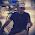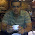Tuesday, September 18, 2012

grep vs awk : 10 examples of pattern search

"grep vs awk" does not mean comparison between awk and grep because there is no comparison to awk whatsoever from grep.  awk is a highly powerful programming language whereas grep is just a filtration tool. However, many of the things which is done using grep and few more commands can be done using a simple awk command. In this article, we will see the awk altenative for the frequently used grep commands.

Let us consisder a file with the following contents:
```\$ cat file
Unix
Linux
Solaris
AIX
Ubuntu
Unix
```
1. To search for the pattern Linux in file:
```\$ grep Linux file
Linux
```
The most simple grep statement. grep followed by pattern name searches for all the lines matching the pattern in the file.
```\$ awk '/Linux/' file
Linux
```
Using awk, the same thing can be done by placing the pattern within slashes.

2. To do a case-insensitive search for 'Linux':
```\$ grep -i linux file
Linux
```
-i option in grep does a case-insensitive search.
```\$ awk '/linux/' IGNORECASE=1 file
Linux
```
IGNORECASE is a special built-in variable present in GNU awk/gawk. When it is set to a non-zero value, it does a case insentive search.

3. To count total number of lines containing the pattern 'Unix':
```\$ grep -c Unix file
2```
The -c option of grep does the total count of the patterns present in a file. Keep in mind, this does a line count. So, if a pattern is present twice in a line, it still will be counted as one.
```\$ awk '/Unix/{x++;}END{print x}' file
2
```
A variable x is incremented when the pattern Unix is encountered. And once the end of the file is reached(END), the count is printed.

4. To get the list of filenames containing the pattern 'AIX':
```\$ grep -l AIX file*
file
```
-l option of grep does not print the pattern. It just prints the filename containing the pattern. This example also shows grep can search in multiple files.
```\$ awk '/AIX/{print FILENAME;nextfile}' file*
file
```
awk has a special variable FILENAME which contains the name of the file which is currently being worked upon. So, the FILENAME is printed everytime the pattern is encountered. nextfile is the awk command to quit the current file and start working on a new file. Without this command, if the pattern is present twice in a file, the file name will also get printed twice.

5. To print the line number along with the pattern matching line:
```\$ grep -n Unix file
1:Unix
7:Unix
```
-n option of grep is used to print the line number along with the pattern matching line.
```\$ awk '/Unix/{print NR":"\$0}' file
1:Unix
7:Unix
```
awk has a special built in variable NR which contains the line number of the particular line being processed.

6. To search for multiple patterns 'Linux' & 'Solaris' in the file:
```\$ grep -E 'Linux|Solaris' file
Linux
Solaris
```
-E option is used for extended regular expressions. Using -E, multiple patterns can be provided to search.
```\$ awk '/Linux|Solaris/' file
Linux
Solaris
```
No special option is needed for the awk command. awk, by default, can accept multiple patterns using the pipe.

7. To do a negative search for a pattern 'Linux':
```\$ grep -v Linux file
Unix
AIX
Ubuntu
Unix
```
-v option of grep gives the inverse result.i.e, it prints all lines not containing the search pattern.
```\$ awk '!/Linux/' file
Unix
AIX
Ubuntu
Unix
```
By giving the exclamation before the pattern, all the lines not containg the pattern is printed.

8. To print a line next to the pattern match and also the line containing the pattern 'Linux':
```\$ grep -A1 Linux file
Linux
Solaris
```
-A 1 prints one line which is next to the line containing the pattern. The line containing the pattern also gets printed since it the default grep behavior.
```\$ awk '/Linux/{print;getline;print}' file
Linux
Solaris
```
'print;getline;print' => print prints the current line which contains the pattern. getline gets next line from buffer and stores in \$0. print prints the line present in \$0. In this way, lines next to the pattern can also be printed.

9. To print a line before the pattern match and also the line containing the pattern 'Solaris':
```\$ grep -B1 Solaris file
Linux
Solaris
```
-B is for printing lines before the pattern.
```\$ awk '/Solaris/{print x;print;next}{x=\$0;}' file
Linux
Solaris
```
Every line read is stored in the variable x. Hence, when the pattern matches, x contains the previous line. And hence, by printing the \$0 and x, the current line and the previous line gets printed.

10. To print the previous, the pattern matching line and next line:
```\$ grep -C1 Solaris file
Linux
Solaris
AIX
```
-C is to print both lines above and below pattern.
```\$ awk '/Solaris/{print x;print;getline;print;next}{x=\$0;}' file
Linux
Solaris
AIX
```
Just the combinations of solutions given for above and below.1.Do you realize that the sample file you list at the top does not actually contain the word "Linux", therefore many of the examples are incorrect. Otherwise, good article.

1.Oops, got deleted somehow during formatting the post..Thanks a lot for for letting me know..updated it..

2.I love this blog!

1.Thanks Mike!!!

3.Very Good Blog. Thank You !

4.Many of the examples used in this page are incorrect, do not follow the advice given here. For example the correct awk answer for "To print a line next to the pattern match and also the line containing the pattern 'Linux':" is NOT "awk '/Linux/{print;getline;print}' file", instead it's "awk '/Linux/{c=2} c&&c--' file". The former will cause you all sorts of headaches in MANY situations including if you try to enhance it in future to print N subsequent lines instead of 1, or if you want to run it on multiple input files since the "getline" will jump over the first line of the next file if the pattern matched is the last line of the current file.

1.The solution provided was to address a particular question, not meant to be a generic one, and the provided solution addresses it.

5.6.7.